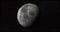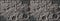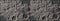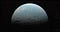# 2. Collect data.

`import urllib.requestelevation_file=“Lunar_LRO_LOLA_Global_LDEM_118m_Mar2014.tif”url=“http://planetarymaps.usgs.gov/mosaic/Lunar_LRO_LOLA_Global_LDEM_118m_Mar2014.tif"urllib.request.urlretrieve(url, elevation_file)`
`from plotoptix.install import download_file_from_google_drivecolor_file=“moon_color_10k_8bit.tif”fid=“1gJeVic597BUAkpz1GgCYRMJVninKEDKB”download_file_from_google_drive(fid, color_file)`
`import urllib.requeststarmap_file=“starmap_16k.tif”url=“https://svs.gsfc.nasa.gov/vis/a000000/a003800/a003895/starmap_16k.tif"urllib.request.urlretrieve(url, starmap_file)`

# 4. Prepare data.

`from plotoptix.utils import read_imageelev_src = read_image(elevation_file)`
`import numpy as npelev_src.dtype = np.int16scale = 1. / np.iinfo(np.int16).maxdownscale = 20h = elev_src.shape // downscalew = elev_src.shape // downscaleelevation = elev_src.reshape(1, h, downscale, w, downscale).mean(4, dtype=np.float32).mean(2, dtype=np.float32).reshape(h, w)elevation *= scale # in-place, save memory!`
`import matplotlib.pyplot as pltplt.figure(1, figsize=(9.5, 5.5))plt.tight_layout()imgplot = plt.imshow(elevation)`
`import cv2color_src = cv2.imread(color_file)# convert from BGR to RGB, 32bit floating point:color_src = color_src[..., ::-1].astype(np.float32)# adjust brightness range, to your taste:color_src = 0.2 + (0.75 / 255) * color_src`
`color_map = cv2.resize(color_src, dsize=elevation.shape[::-1], interpolation=cv2.INTER_CUBIC).astype(np.float32)# clamp out-of-range interpolation results:_ = np.clip(color_map, 0, 1, out=color_map)`
`plt.figure(2, figsize=(9.5, 5.5))plt.tight_layout()color_plot = plt.imshow(color_src)`
`# target for downscaling from the original 16k width:star_map_width = 8192star_src = cv2.imread(starmap_file)star_src = star_src[..., ::-1].astype(np.float32)star_src *= 1 / 255if star_map_width < star_src.shape:    star_map_height = int(star_src.shape * star_map_width / star_src.shape)    star_map_size = (star_map_width, star_map_height)    star_map = cv2.resize(star_src, dsize=star_map_size, interpolation=cv2.INTER_CUBIC).astype(np.float32)    np.clip(star_map, 0, 1, out=star_map) # clamp out-of-range interpolation resultselse:    star_map = star_srcplt.figure(3, figsize=(9.5, 5.5))plt.tight_layout()# plot sqrt to boost faint lights:color_plot = plt.imshow(np.sqrt(star_map))`

# 5. Setup ray tracing.The Moon, ray-traced in natural colors.
`from plotoptix import TkOptiXfrom plotoptix.utils import make_color_2drt = TkOptiX()# accumulate up to 50 frames to remove the noise:rt.set_param(max_accumulation_frames=50)# add tonal correction:exposure = 0.9; gamma = 2.2rt.set_float(“tonemap_exposure”, exposure)rt.set_float(“tonemap_igamma”, 1 / gamma)rt.add_postproc(“Gamma”)# star map in the background:rt.set_background_mode(“TextureEnvironment”)rt.set_background(star_map, gamma=gamma)# setup camera with a good point of view:rt.setup_camera("cam1", cam_type="DoF",                eye=[-13, -22, 3],                target=[0, 0, 0],                up=[0, 0.9, 0.5],                aperture_radius=0.06,                aperture_fract=0.2,                focal_scale=0.62,                fov=55)# add the Sun to light up the scene:rt.setup_light("sun", pos=[-50, 0, 0], color=60, radius=6)`
`def sphere(u, v, r):    sinu = np.sin(u)    x = sinu * np.cos(v)    y = sinu * np.sin(v)    z = np.cos(u)    return r * np.array([x, y, z], dtype=np.float32)iu = np.arange(elevation.shape, dtype=np.float32)iv = np.arange(elevation.shape, dtype=np.float32)V, U = np.meshgrid(iv, iu)U *= np.pi / elevation.shapeV *= 2 * np.pi / elevation.shapermin = np.min(elevation)rmax = np.max(elevation)rv = rmax - rmin# rescale "in place": 0.98 + (0.02/rv)*(elevation+rmin)elevation += rminelevation *= 0.02 / rvelevation += 0.98elevation *= 10 # max radiusS = sphere(U, V, elevation).T`
`rt.set_surface(    “moon”, S,    c=np.swapaxes(make_color_2d(color_map, gamma=gamma), 0, 1),    wrap_v=True,    make_normals=True)`
`rt.start()`Close-up with the mesh normals interpolation off (left) and on (right).
`rt.close()`
`rt.set_data("moon",    mat="diffuse",                # actually, a default material    geom="ParticleSetTextured",   # spherical, textured shape    geom_attr="DisplacedSurface", # try "NormalTilt", see notebooks    pos=[0, 0, 0],    u=[0, 0, 1],    v=[-1, 0, 0],    r=10)`
`from plotoptix.materials import m_diffusefrom plotoptix import RtFormat# first, setup a dummy 2x2 texture:m_diffuse["Textures"]=[        {          "Width": 2, "Height": 2,          "DataArray": 2*2*4 * [1.0], # 2x2 RGBA, flat list          "Format": RtFormat.Float4.value        }      ]rt.update_material("diffuse", m_diffuse)# then, update the dummy texture with the actual content:color_map=make_color_2d(color_map, gamma=gamma,                        channel_order="RGBA")rt.update_material_texture("diffuse", color_map)`
`rt.start()`
`rmin = np.min(elevation)rmax = np.max(elevation)rv = rmax - rmin# rescale “in place”: 0.98 + (0.02/rv)*(elevation+rmin)elevation += rminelevation *= 0.02 / rvelevation += 0.98rt.set_displacement("moon", elevation,    mapping="Spherical",    displacement="DisplacedSurface", # try "NormalTilt"    refresh=True)`Maximum level of details I got on a 6GB GPU with the mesh (left) and the displacement map (right) approach.
`rt.close()`

# 6. Summary.Elevation mapped to color, RdYlBu palette.

Written by

## Robert Sulej

#### Generative and machine learning art. Data science. Particle physics background.

Welcome to a place where words matter. On Medium, smart voices and original ideas take center stage - with no ads in sight. Watch
Follow all the topics you care about, and we’ll deliver the best stories for you to your homepage and inbox. Explore
Get unlimited access to the best stories on Medium — and support writers while you’re at it. Just \$5/month. Upgrade